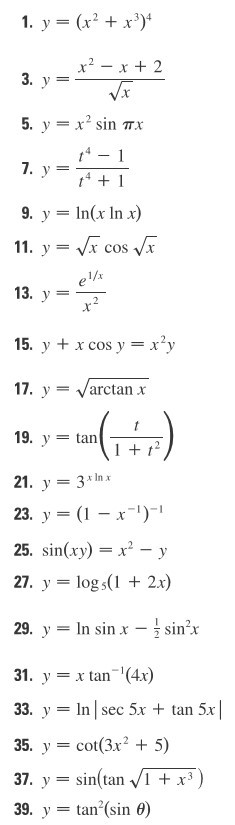# 1. У(x2 + x3)49. Уln(x ln x)1 + t21. У 3x1n х23, y = (1-x-1)-25. sin(xy)-x2-y27. y-logs 2x)29. y--In sin x--sin-х1. yan (4x)33. yInsec 5x + tan 5x35, y = cot(3x2 + 5)37. y=sin(tan-/I +x3

Question
2 views

Differentiation#35help_outlineImage Transcriptionclose1. У (x2 + x3)4 9. У ln(x ln x) 1 + t 21. У 3x1n х 23, y = (1-x-1)- 25. sin(xy)-x2-y 27. y-logs 2x) 29. y--In sin x--sin-х 1. yan (4x) 33. yInsec 5x + tan 5x 35, y = cot(3x2 + 5) 37. y=sin(tan-/I +x3 fullscreen
check_circle

Step 1

We have

Step 2

Formula used:

Step 3

Now differentiate y with respect ...

### Want to see the full answer?

See Solution

#### Want to see this answer and more?

Solutions are written by subject experts who are available 24/7. Questions are typically answered within 1 hour.*

See Solution
*Response times may vary by subject and question.
Tagged in

### Calculus